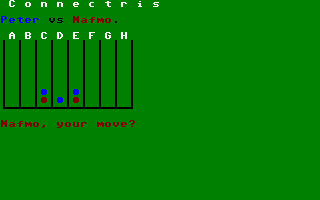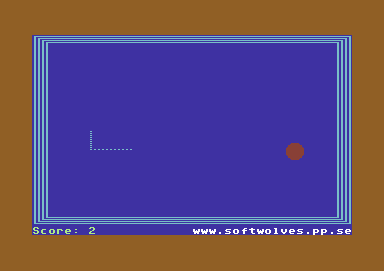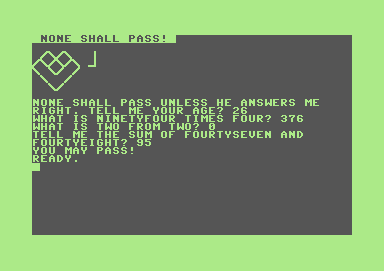# My entries to Minigame 2002

Here are my three simple entries in the Minigame 2002. The task was to write a game for an eight-bit computer in a maximum of one (1) kilobyte. Since my attempts at writing games in assembler failed miserably, these three entries are all written in BASIC.

The three programs described on this page are released according to the GNU General Public License, version 2.

I have also written an article about the event for ABC-Bladet the magazine for members of the Swedish computer club ABC-Klubben.

To those of you using text based browsers: Due to the use of CSS these pages do not display correctly in text based browsers. Links and W3M are the ones that work best, but even they displaya the line numbers in the wrong place.

## Connectris### Instructions

This is an implementation of the game Connectris, an idea I got from the wonderful on-line gaming website It's Your Turn. It's a two player game which combines the well-known Connect Four game and the ever-popular Tetris.

The two players, blue and red, take turns in dropping their "chips" into the play field. You can only select which column to place your chip in, it will always locate itself at the bottommost free row. The player that first get four chips in a row, either horizontal, vertical or diagonal, you win.

The twist is that whenever the bottommost row is completely filled, it gets removed from the game, and all chips are shifted one step downwards!

### Requirements

This game plays in the Commodore C128 80 column mode, and is written entirely in BASIC 7.0.

If started from 40 column mode, it will prompt you to switch modes, and wait for a key to be pressed. If started from anything else than a C128, it will display an error message and exit.

I got the question why this game is running in the C128's 80 column mode when it is not even using 40 columns. There are two reasons: The first is that I can mix lower/upper case letters (the dialogue with the user) with upper case letters/graphics (the game board), the second is that the game speed is double in 2MHz compared to 1MHz, even though it is way too slow... :-/ (If run with a SuperCPU it operates at an acceptable speed, however...)

The program can be fetched here.

Commented source code listing
Entry point
1
`GOTO90`
Select colours and print the title
2
`COLOR6,6:`
Green background
`COLOR5,2:`
White text
`PRINT"{clear}{ct b}{ct n} C o n n e c t r i s {130}":`
`RETURN`
Write who are playing (at the top of each screen)
3
```PRINT"{down}{light blue}{ct n}"a\$" {black}vs {red}"
b\$"{black}.{down}":```
`RETURN`
Print the board
10
`PRINT"{142}{white} a b c d e f g h`
Column labels
11
`FORy=.TO7:`
` FORx=.TO7:`
`  PRINT"{black}{sh -}";:`
Frame
`  COLOR5,p(x,y):`
The board contains colour data
`  PRINT"●";:`
A circle
` NEXT:`
` PRINT"{black}{sh -}":`
`NEXT`
12
```PRINT"{cm z}{sh asterisk}{cm e}{sh asterisk}{cm e}{sh asterisk}
{cm e}{sh asterisk}{cm e}{sh asterisk}{cm e}{sh asterisk}{cm e}
{sh asterisk}{cm e}{sh asterisk}{cm x}":```
Lower frame
`RETURN`
Retrieve a move from the player
20
`DO:`
` DO:`
`  COLOR5,c:`
C = player's colour
`  PRINT"{down}{ct n}"c\$", your move? {142}{black}";:`
C\$ = player's name
`  GETKEYx\$:`
`  x=ASC(x\$)-65:`
Translate A-H to column numbers
`  PRINTx\$:`
` LOOPWHILEx<.ORx>7:`
Check that the column is valid
`LOOPWHILEp(x,.)<>6:`
Check that there is place left (=6=green)
`PRINT"ok`
21
`FORy=7TO.STEP-1:`
Find the lowest free spot
` IFp(x,y)<>6THEN`
`  NEXT`
22
`p(x,y)=c:`
Drop the disc
`FORx=.TO7:`
Is the lowest row full?
` IFp(x,7)<>6THEN`
`  NEXT:`
`  GOSUB30:`
Yes! Empty it
`  RETURN`
Cannot win now
23
`GOSUB40:`
Check winning position
`RETURN`
Remove lowest row and shift everything down one row
30
`FORx=.TO7:`
` FORy=7TO1STEP-1:`
`  p(x,y)=p(x,y-1):`
` NEXT:`
` p(x,.)=6:`
6=green indicates an empty spot
`NEXT:`
`RETURN`
Check winning position
40
`FORy=.TO7:`
` FORx=.TO4:`
`  IFp(x,y)+p(x+1,y)+p(x+2,y)+p(x+3,y)=c*4THEN50`
Horizontal winning position
41
` NEXT:`
`NEXT:`
`FORx=.TO7:`
` FORy=.TO4:`
`  IFx<5THEN`
```   IFp(x,y)+p(x,y+1)+p(x,y+2)+p(x,y+3)=c*4OR
p(x,y)+p(x+1,y+1)+p(x+2,y+2)+p(x+3,y+3)=c*4THEN50```
Vertical or diagonal winning position
42
`  IFx>2THEN`
`   IFp(x,y)+p(x-1,y+1)+p(x-2,y+2)+p(x-3,y+3)=c*4THEN50`
Diagonal winning position
43
` NEXT:`
`NEXT:`
`RETURN`
Say who won
50
`GOSUB2:`
Title line
`COLOR5,c:`
Player's colour
`PRINT"{down}{ct n}You win, "c\$:`
`PRINT:`
`GOSUB10:`
Print the board
`GETKEYz\$:`
`RUN`
Restart
60
`PRINT"{down}{ct n}What's your name, "n\$;:`
`INPUT" player";c\$:`
`RETURN`
Startup routine
90
`IFINT(.1+.9)<>1THEN`
Test BASIC 7
` PRINT"{reverse on} c128 mode ":`
` END`
92
`IFRGR(0)<>5THEN`
Check 80 column mode
` PRINT"{reverse on} switch to 80 columns ":`
` GRAPHIC5:`
Switch to 80 column mode
` GETKEYz\$`
100
`FAST:`
Double speed...
`GOSUB2`
Title line
120
`n\$="blue":`
Get name of blue player
`DO:`
` COLOR5,15:`
` GOSUB60:`
`LOOPWHILEc\$="":`
`a\$=c\$`
130
`n\$="red":`
Get name of red player
`DO:`
`COLOR5,3:`
`GOSUB60:`
`LOOPWHILEc\$=""ORc\$=a\$:`
`b\$=c\$`
140
`DIMp(7,7):`
Board
`FORx=.TO7:`
Empty board
` FORy=.TO7:`
(fill with green discs)
`  p(x,y)=6:`
6=green=background=empty
` NEXT:`
`NEXT`
Huvudslinga
200
`c=3:`
3=red player (blue starts)
`DO:`
` ONc/8+1GOSUB210,220:`
Switch current player
` GOSUB2:`
Title line
` GOSUB3:`
Who are playing
` GOSUB10:`
Draw board
` GOSUB20:`
Fetch a move
`LOOP`
Pick blue as current player
210
`c=15:`
`c\$=a\$:`
`RETURN`
Pick red as current player
220
`c=3:`
`c\$=b\$:`
`RETURN`

## Maggot### Instructions

This is the famous "Masken" ("Snake" in English). I was first exposed to this game idea with Luxor's Masken for the ABC-80. You are a worm (or maggot, in this case), and your aim is to eat as many targets as possible. Whenever you eat a target, the worm grows. If you run into the walls or yourself, the game is over.

The game is controlled with the numbers on the numerical keypad: 8 for up, 4 for left, 6 for right and 2 for down. Any other key makes the worm stop, which means instant Game Over!

### Requirements

This games plays in the Commodore C128 40 column mode, and is written entirely in BASIC 7.0.

If started from 80 column mode, it will automatically switch over to 40 column mode for you.

Version 1.1 - 2002-08-13:
• Fixed bug that would cause the game to fail on first run. Seems like DEFined functions will not survive the program relocation done by the GRAPHIC command.
• Fixed a spelling mistake.

Maggot is a variant of the famous Masken (Snake) theme. I first saw Masken on a Luxor ABC-80, and that game was written entirely in BASIC. Now, ABC BASIC is fast, contrary to Commodore's BASIC, so I thought it would be an interesting challenge to write a version of Masken in Commodore BASIC, in graphics mode, which at least was not unplayingly slow. It succeeded partly.

The program can be fetched here. Observera! VICE version 1.9 and 1.10 are buggy and cannot handle this program properly. It works in version 1.8.

Commented source code listing
Entry point
0
`GRAPHIC1,1:`
Switch to graphics mode
`FAST:`
Speed up initialization (and blank the screen)
`DEFFNr(x)=INT(RND(.)*x):`
Create integer random numbers in [0,X)
`DEFFNn(x)=x+1+zz*(x=z):`
Increase X wrapping at Z
`GOTO10`
Go to main initialization
Move the maggot
5
`LOCATEx(h),y(h):`
Move the graphics pointer to where we are heading
`IFRDOT(2)THEN100`
If there is anything there we die
6
`GETa\$:`
See if we pressed a key
`IFa\$<>""THENGOSUB9`
If we did, we change direction
7
`DRAW1:`
Move the maggot
`DRAW.,x(t),y(t):`
`t=FNn(t):`
Calculate next index for the tail
`IFti>pTHENGOSUB93`
Time to move the target?
8
`RETURN`
Change direction
9
`r=4*((a\$="4")-(a\$="6")):`
4=left, 9=right
`d=2*((a\$="8")-(a\$="2")):`
8=up, 2=down
`RETURN`
Initialize the game
10
`COLOR0,7:`
Blue background
`COLOR1,4:`
Cyan graphics
`COLOR4,9:`
Orange frame
`WIDTH2`
All graphics two pixels wide
11
`CIRCLE,40,40,8:`
Target is a filled circle
`PAINT,40,40:`
`SSHAPEt\$,32,32,55,53:`
Save as a sprite
`GRAPHIC1,1:`
Empty the screen
`SPRSAVt\$,1`
Define the circle as sprite image 1
12
`FORi=1TO7STEP2:`
` BOX1,i*2,i,319-i*2,189-i:`
Draw frames around the screen
`NEXT:`
`COLOR1,14:`
Light green colour
`CHAR,0,24,"{ct n}Score: 0`
Score counter
13
`COLOR1,2:`
White colour
`CHAR,20,24,"{ct n}www.softwolves.pp.se":`
`COLOR1,4`
The maggot is cyan
20
`z=1024:`
Maximum length is 1024
`zz=z+1:`
Help variable for FNN
`DIMx(z),y(z):`
`FORi=.TO9:`
` x(i)=18+i*4:`
Start position (100,18) - (100,36)
` y(i)=100:`
` DRAW,x(i),100:`
Paint
`NEXT:`
`h=9:`
`t=.`
Tail at index 0
21
`GOSUB93:`
Prepare first target
`SLOW:`
Show screen
`r=4:`
Moving to the right
`d=.`
Not up
Huvudslinga
30
`DO:`
` DO:`
Loop for moving left/right
`  o=h:`
`  h=FNn(h):`
`  x(h)=x(o)+r:`
Move right/left
`  IFx(h)<15ORx(h)>304THEN100`
Check screen borders
31
`  y(h)=y(o):`
`  GOSUB5`
Move
32
` LOOPWHILEr`
40
` DO:`
Loop for moving up/down
`  o=h:`
`  h=FNn(h):`
`  y(h)=y(o)+d:`
Move up/down
`  IFy(h)<8ORy(h)>181THEN100`
41
`  x(h)=x(o):`
`  GOSUB5`
42
` LOOPWHILEd:`
`LOOP`
Hit registered
90
`COLLISION2:`
Deactivate detection
`SPRITE1,1,1:`
Make the target black to indicate hit
`s=s+BUMP(2):`
Increase the score and nullify the collision
`COLOR1,14:`
`CHAR,6,24,STR\$(s):`
Print the score
`COLOR1,4`
91
`e=FNr(5)+3:`
Randomize how much to increase the maggot's length with
`DO:`
` o=h:`
` h=FNn(h):`
` IFh=tTHEN`
Have we reached the maximum?
`  h=o:`
If so we stop increasing
`  EXIT:`
` ELSE`
`  x(h)=x(o):`
Otherwise we do increase its length
`  y(h)=y(o):`
`  e=e-1:`
`LOOPWHILEe`
92
`SPRITE1,.:`
Hide target
`MOVSPR1,.,.:`
Move it away from the screen
`p=30+FNr(60):`
Randomize time for return (0.5-1.5s)
`GOTO94`
Clear the timer
Randomize a target
93
`x=FNr(269)+42:`
Randomize coordinates for the target
`y=FNr(156)+59:`
`MOVSPR1,x,y:`
Move the target there
`COLLISION2,90:`
Enable hit detection
`SPRITE1,1,FNr(5)+2,.,.,.,.:`
Enable the sprite
`p=360+FNr(360)`
Randomize time until the target is moved (6-12s)
94
`ti\$="000000":`
Clear the timer
`RETURN`
Game over
100
`CHAR,18,24,"{ct n}Game over! Play again?":`
Tell player the game is over
`GETKEYz\$:`
`IFz\$="y"THENRUN`
Restart if we want to
101
`GRAPHIC.:`
Restore text mode
`SPRITE1,.:`
Remove target
`COLOR.,1:`
Black background
`COLOR4,1:`
Black border
`PRINT"{clear}{ct n}{white}You caught"s"target";`
Show score
102
`IFs<>1THENPRINT"s";`
(in plural)

## None Shall Pass### Instructions

This is a simple "educational" game, in which you only may pass the horrible ogre (display on-screen as a rather ugly figure-head) if you can answer its quiz correctly. In this case, the quiz is made up of mathematical problems.

First you enter your age, which selects the skill level, and then you are presented with the questions. You must answer all questions correctly to be allowed to pass!

If the questions are too hard, try lying about your age! :)

### Requirements

This game plays on any Commodore 8-bit machine equipped with BASIC version 2 or later. It automatically adapts its output to fit your screen, no matter if it is a VIC 20 or an C128 in 80-column mode.

I'm not really sure if I really want to classify this as a game... I got the idea one night after going to bed and couldn't get it out of my head, so I went up and wrote the game down on my C128. What made me send it to the competition was the fact that I adapted the program to run on any Commodore machine with BASIC 2. To be sure, I tested it on PET 3032, VIC-20, C64 and C128... I was happy to see that it didn't finish last... (I wonder who was, ehm, kind enough to give it a ten out of ten score?) The idea of three questions to pass a bridge I got from the movie Monthy Pythons and the Holy Grail.

The program can be fetched here.

Commented source code listing
Entry point
0
`DEFFNr(r)=RND(.)*r+1:`
Random number generator
`GOTO10`
Go to the main program
Line break a text and print it
1
`IFLEN(z\$)<=wTHEN`
Does the text fit on the line?
` PRINTz\$;:`
If so print it directly
` RETURN`
2
`FORi=wTO.STEP-1:`
` IFMID\$(z\$,i,1)<>" "THENNEXT`
Find a strategic space character
3
`PRINTLEFT\$(z\$,i):`
Print up to the space
`z\$=MID\$(z\$,i+1):`
Cut the string
`GOTO1`
And start over
Main program
10
`PRINT"{clear}{down}{left}";:`
Figure out the width of the screen
`w=POS(.)`
11
`PRINT"{home}{reverse on} none shall pass! {down}":`
Print the title
`PRINT" /\/\  {cm m}":`
`PRINT"/\/\/\ {sh @}":`
`PRINT"\ \/ /":`
`PRINT" \  /":`
`PRINT"  \/{down}`
12
`DIMa\$(99):`
Numerals
`FORi=1TO19:`
Get words for 1-19
` READa\$(i):`
`NEXT:`
`FORi=20TO90STEP10:`
` READa\$(i):`
Get tens
` FORj=1TO9:`
`  a\$(i+j)=a\$(i)+a\$(j):`
Construct numerals for each ten
` NEXT:`
`NEXT`
20
`z\$="none shall pass unless he answers me right. tell me your age":`
`GOSUB1`
21
`INPUTa%:`
Get age, i.e difficulty level
`IFa%<2ORa%>130THEN90`
Check if the answer is reasonable
22
`m=10:`
Lowest difficulty level only has single digits
`n=m:`
`IFa%>11THEN`
` m=20:`
Next level has up to
` n=12:`
twelve's multiplcation table
`  IFa%>15THEN`
`   m=99`
Next level has all numbers
23
`y\$="what is ":`
Query text
`IFa%<8THEN30`
Lowest difficulty level does not have multiplication
25
`f1%=FNr(m):`
Question 1
`f2%=FNr(n)`
26
`z\$=y\$+a\$(f1%)+" times "+a\$(f2%):`
Multiplication
`GOSUB1:`
`INPUTp:`
`IFp<>f1%*f2%THEN90`
30
`t1%=FNr(m):`
Question 2
`t2%=FNr(t1%):`
`IFt2%=0THEN30`
Make sure we are not subtracting zero
31
`z\$=y\$+a\$(t2%)+" from "+a\$(t1%):`
`GOSUB1:`
`INPUTs:`
`IFs<>t1%-t2%THEN90`
35
`a1%=FNr(m):`
Question 3
`a2%=FNr(m):`
`IFa1%+a2%=0THEN35`
36
`z\$="tell me the sum of "+a\$(a1%)+" and "+a\$(a2%):`
`GOSUB1:`
`INPUTa:`
`IFa<>a1%+a2%THEN90`
Win
80
`z\$="you may pass!":`
Congratulations, you won!
`GOSUB1:`
`END`
Lose
90
`z\$="that's not correct!":`
You lost
`GOSUB1:`
`END`
Numerals
100
```DATAone,two,three,four,five,six,seven,eight,nine,ten,
eleven,twelve,thirteen,fourteen,fifteen,sixteen,
seventeen,eighteen,nineteen```
101
```DATAtwenty,thirty,fourty,fifty,sixty,seventy,
eighty,ninety```